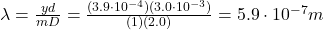## A beam of yellow light is made to pass through two slits that are 3.0 x 10−3 meters apart. On a screen 2.0 meters away from the slits, an in

Question

A beam of yellow light is made to pass through two slits that are 3.0 x 10−3 meters apart. On a screen 2.0 meters away from the slits, an interference pattern appears with bands of light separated by 3.9 x 10−4 meters. What is the wavelength of the light?

3.6 x 10−8 m
5.9 x 10−7 m
6.5 x 10−7 m
3.9 x 10−6 m

in progress 0
3 months 2021-07-30T16:46:14+00:00 1 Answers 12 views 0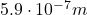Explanation:

The formula to calculate the position of the m-th maximum in the interference pattern produced by the diffraction of light from a double slit is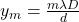where

y is the distance of the maximum from the central bright fringe

m is the order of the maximum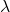is the wavelength of the light

D is the distance of the screen from the slits

d is the distance between the slits

Here we have: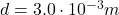(distance between slits)

D = 2.0 m (distance of the screen)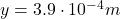is the distance of the 1st maximum after the central maximum from the central fringe (therefore, taking m = 1)

Solving for, we find the wavelength: